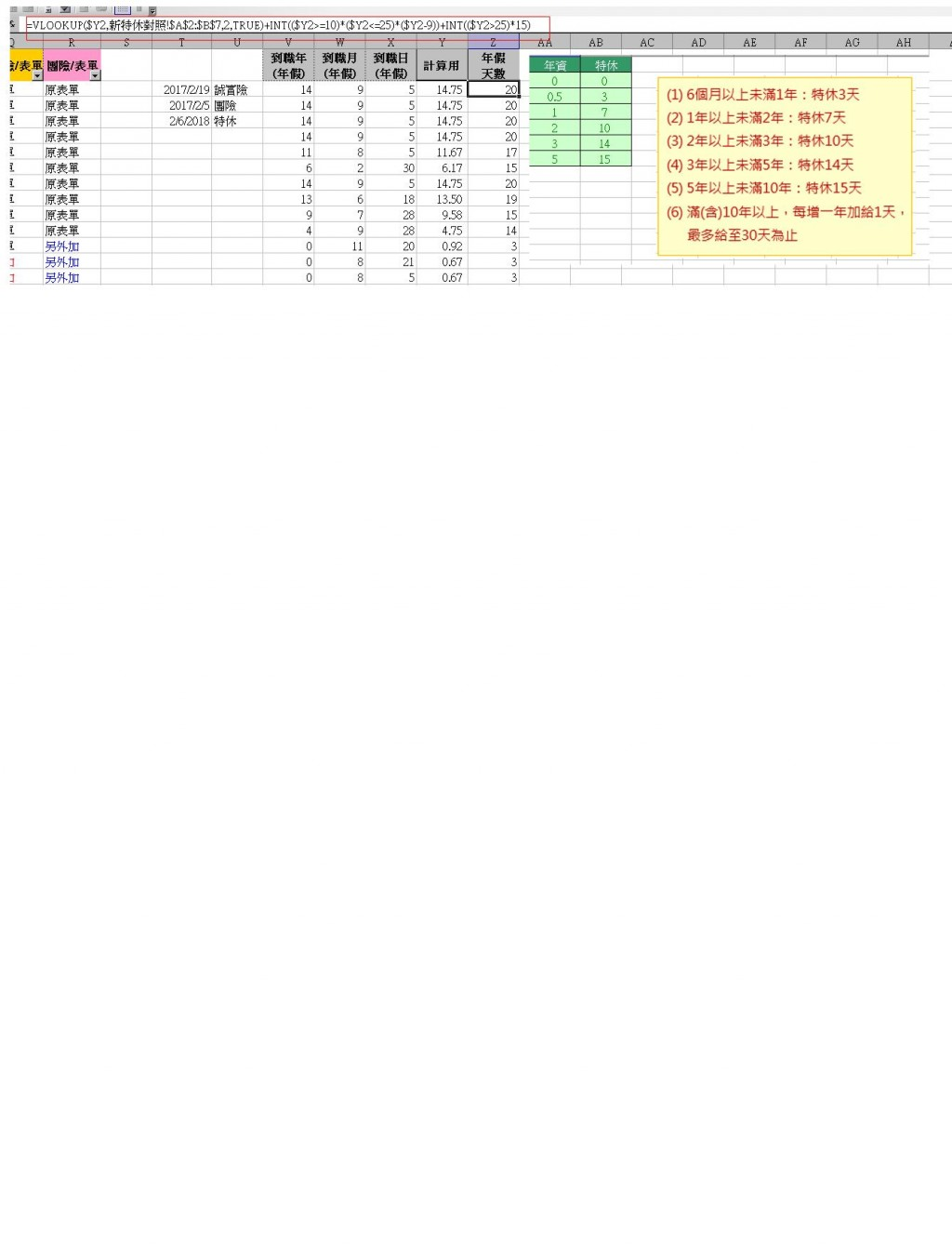0

excel 年假的公式

=VLOOKUP(\$Y2,新特休對照!\$A\$2:\$B\$7,2,true) true 是指?
+INT((\$Y2>=10) 為什麼要>=10
(\$Y2<=25)(\$Y2-9)) 為什麼要>=25 * -9
+INT((\$Y2>25)15) 為什麼要>25 * 再151 個回答

3

VLookUp你可以搜尋一下用法，

10年以上，每滿1年多1天
INT((\$Y2>=10)(\$Y2<=25)(\$Y2-9))

Y2 >= 10 成立，所以是1(不成立是0)
Y2 <= 25 成立，所以是1

INT((\$Y2>25)15)

swhung iT邦新手 5 級 ‧ 2018-02-07 17:28:39 檢舉